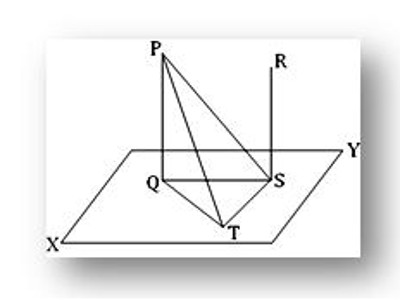Theorem on Parallel Lines and Plane

Theorem: If two straight lines are parallel and if one of them is perpendicular to a plane, then the other is also perpendicular to the same plane. When lines and planes are perpendicular and parallel, they have some interesting properties.

Lines and planes are parallel to one another as in the ordinary geometry: two lines when they lie in one plane and do not intersect, a line and a plane or two planes when they lie in one hyperplane and do not intersect.

Let PQ and RS be two parallel straight lines of which PQ is perpendicular to the plane XY. We are to prove that the straight line RS is also perpendicular to the plane XY.Construction: Let us assume straight line PQ and RS intersect the plane XY at Q and S respectively. Join QS. Evidently, QS lies in the XY plane. Now, through S draw ST perpendicular to QS in the XY plane. Then, join QT, PT, and PS.

Proof: By construction, ST is perpendicular to QS. Therefore, from the right-angled triangle QST, we get,

QT² = QS² + ST² ………………(1)

Since PQ is perpendicular to the plane XY at Q and the straight lines QS and QT lie in the same plane, therefore PQ is perpendicular to both the lines QS and QT. Therefore, from the right-angle PQS, We get,

PS ² = PQ ² + QS ²      ………………(2)

And from the right-angle PQT, we get,

PT² = PQ² + QT² = PQ² + QS² + ST² [using (1)]

or, PT² = PS² + ST² [using (2)]

Therefore, ∠PST = 1 right angle. i.e., ST is perpendicular to PS. But by construction, ST is perpendicular to QT.

Thus, ST is perpendicular to both PS and QS at S. Therefore, ST is perpendicular to the plane PQS, containing the lines PS and QS.

Now, S lies in the plane PQS and RS are parallel to PQ; hence, RS lies in the plane of PQ and PS i.e., in the plane PQS. Since ST is perpendicular to the plane PQS at S and RS lies in this plane, hence ST is perpendicular to RS i.e., RS is perpendicular to ST.

Again, PQ and RS are parallel and ∠PQS = 1 right angle.

Therefore, ∠RSQ = 1 right angle i.e., RS is perpendicular to QS. Therefore, RS is perpendicular to both QS and ST at S; hence, RS is perpendicular to the plane containing QS and ST i.e., perpendicular to the XY.

Information Source: